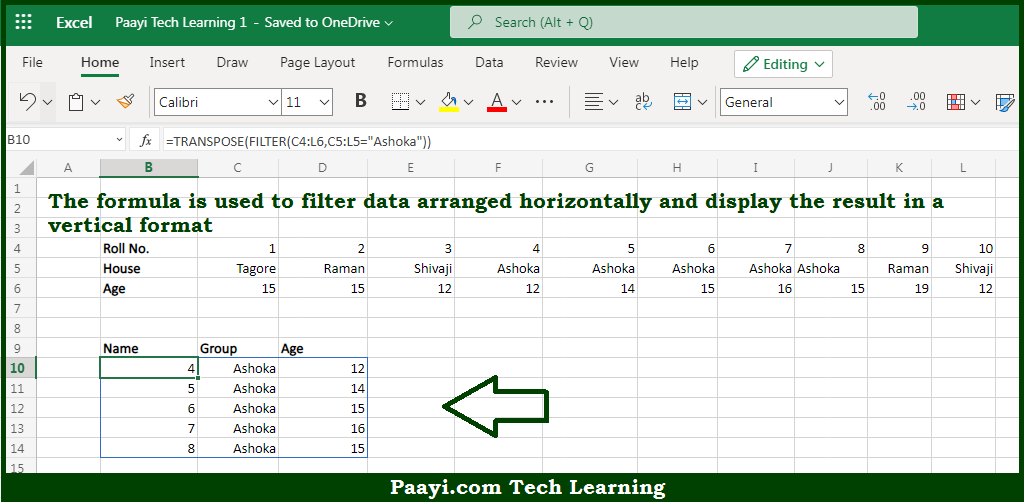# Learn How to Filter and Transpose Horizontal to Vertical in Microsoft Excel

Written by | 0 Comments | 1132 Views

In this article, you will learn how to evaluate things in Dynamic Arrays with formulas in Microsoft Excel using a single/combination(s) of functions. You will also know How to Filter and Transpose Horizontal to Vertical and see the generic formula.

How to Filter and Transpose Horizontal to Vertical in Microsoft Excel

The main purpose of this formula is to filter data arranged horizontally and display the output in a vertical format. Here we will learn how to filter and transpose horizontal to vertical from a given data range in the workbook in Microsoft Excel. That implies, with the help of a formula based on the FILTER and TRANSPOSE function you can able to filter data arranged horizontally and display the result in a vertical format. So, with the help of this formula, you can be able to vertical from a given data range in the workbook in Microsoft Excel.

General Formula to Filter and Transpose Horizontal to Vertical

=TRANSPOSE(FILTER(data,logic))

The Explanation for the Filter and Transpose Horizontal to VerticalSo we know that with the help of the given formula above you can able to filter data arranged horizontally and display the output in a vertical format. Here we will learn how to filter and transpose horizontal to vertical from a given data range in the workbook in Microsoft Excel. As we know that the  FILTER function can be used to extract data arranged vertically in rows or horizontally in columns. Besides, that FILTER function will return the matching data in the same orientation. So, with the help of this formula, you can able to filter data arranged horizontally and display the output in a vertical format. Here we will learn how to filter and transpose horizontal to vertical from a given data range in the workbook in Microsoft Excel.